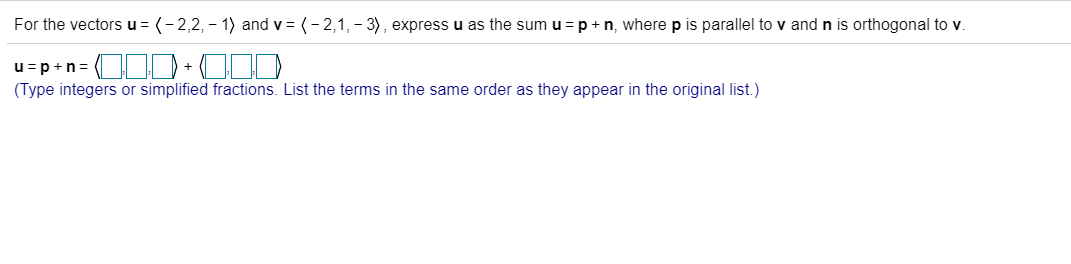# For the vectors u = (-2,2, -1) and v(-2,1, 3), expressu as the sum u =p n, where p is parallel to v and n is orthogonal to v.u pn+(Type integers or simplified fractions. List the terms in the same order as they appear in the original list.)

Question
1 viewshelp_outlineImage TranscriptioncloseFor the vectors u = (-2,2, -1) and v (-2,1, 3), expressu as the sum u =p n, where p is parallel to v and n is orthogonal to v. u pn + (Type integers or simplified fractions. List the terms in the same order as they appear in the original list.) fullscreen
check_circle

Step 1

To find the orthogonal decomposition of the vector u along and perpendicular to v

Step 2

### Want to see the full answer?

See Solution

#### Want to see this answer and more?

Solutions are written by subject experts who are available 24/7. Questions are typically answered within 1 hour.*

See Solution
*Response times may vary by subject and question.
Tagged in

### Other